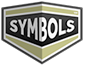# Triangle

#### This page is about the meaning, origin and characteristic of the symbol, emblem, seal, sign, logo or flag: Triangle.

Rate this symbol:(5.00 / 3 votes)A triangle is one of the basic shapes in geometry: a polygon with three corners or vertices and three sides or edges which are line segments.

In Euclidean geometry any three points, when non-collinear, determine a unique triangle and a unique plane (i.e. a two-dimensional Euclidean space).

Triangles are assumed to be two-dimensional plane figures, unless the context provides otherwise (see Non-planar triangles, below). In rigorous treatments, a triangle is therefore called a 2-simplex (see also Polytope). Elementary facts about triangles were presented by Euclid in books 1–4 of his Elements, around 300 BC.

The measures of the interior angles of the triangle always add up to 180 degrees (same color to point out they are equal).

The measures of the interior angles of a triangle in Euclidean space always add up to 180 degrees. This allows determination of the measure of the third angle of any triangle given the measure of two angles. An exterior angle of a triangle is an angle that is a linear pair (and hence supplementary) to an interior angle. The measure of an exterior angle of a triangle is equal to the sum of the measures of the two interior angles that are not adjacent to it; this is the exterior angle theorem. The sum of the measures of the three exterior angles (one for each vertex) of any triangle is 360 degrees.

1,264 Views

Graphical characteristics:
Symmetric, Closed shape, Monochrome, Contains straight lines, Has no crossing lines.

Category: Geometry Symbols.## More symbols in Geometry Symbols:

Geometry (Ancient Greek: γεωμετρία; geo- "earth", -metron "measurement") is a branch of mathematics concerned with questions of shape, size, relative position of figures, and the properties of space.… read more »

### Citation

#### Use the citation below to add this symbol to your bibliography:

Style:MLAChicagoAPA

"Triangle." Symbols.com. STANDS4 LLC, 2021. Web. 25 Jan. 2021. <https://www.symbols.com/symbol/triangle>.

## Graphical index

### Use our unique search feature to find a symbol based on its various graphical characteristics:

• Symmetry:
• Shape:
• Colors:
• Curveness:
• Crossing: